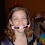## Monday, August 13, 2007

### And I Will Raise You Up

Given that x^(x^(x^(x^(x^(x^(x^(x^(x...)))...) = 2, solve for x.

1.Limit X^(infinity)=2 X-> 1

In other words, the infinate root of 2 is a hair larger than 1.

2.Mr Don, of course, is wrong: there's no particular reason that the exponent need by infinite.

Indeed, if x^(x^(x^(x^(x^(x^(x^(x^(x...)))...) is going to have any meaning at all, then we can solve the equation by substitution:

x^(x^(x^(x^(x^(x^(x^(x^(x...)))...) = 2

x^(x^(x^(x^(x^(x^(x^(x^(x^(x...)))...)) = x^2

so x^2 = 2, and x=\pm\sqrt{2}.

But, of course, \sqrt{2} > 1, so the limit goes to \infty if taken in the usual metric, whereas negative numbers aren't easily raised to irrational exponents (the function is multivalued without a good choice for what value it should take). Which is to say: x ought to equal \sqrt{2}, but only with a careful definition for the infinite exponent.

3.I'm not sure I want to get involved in this debate.

4.Oh. With such a lucid explanation, how can anyone dispute the correct answer?

5.haha, you know he's hardcore if he writes his answer in pseudo-latex code. mr. don, I detected some sarcasm...

I'm sorry I was out of town for this one, I would have liked to weigh in. theo is right.

x^(x^(x...))...) = 2
x^(x^(x^(x...))...) = x^2

but the left sides of those two equations are identical, so x^2 = 2. thus, x = sqrt(2).

Leave your answer or, if you want to post a question of your own, send me an e-mail. Look in the about section to find my e-mail address. If it's new, I'll post it soon.

Please don't leave spam or 'Awesome blog, come visit mine' messages. I'll delete them soon after.

Enter your Email and join hundreds of others who get their Question of the Day sent right to their mailbox

The Lamplight Manor Puzz 3-D